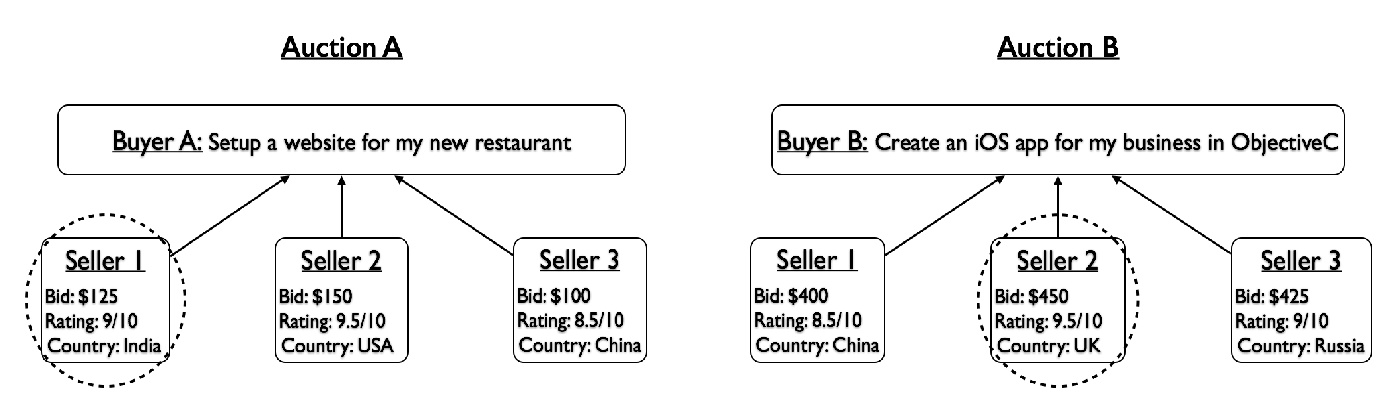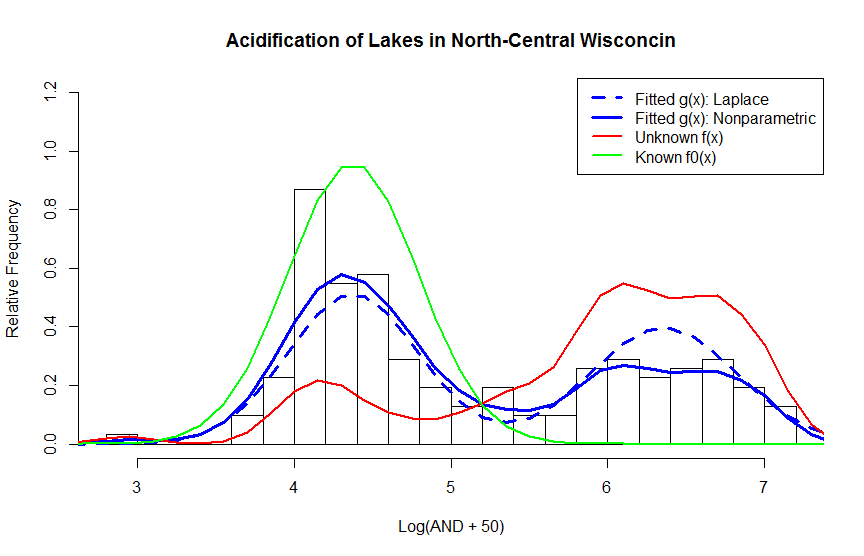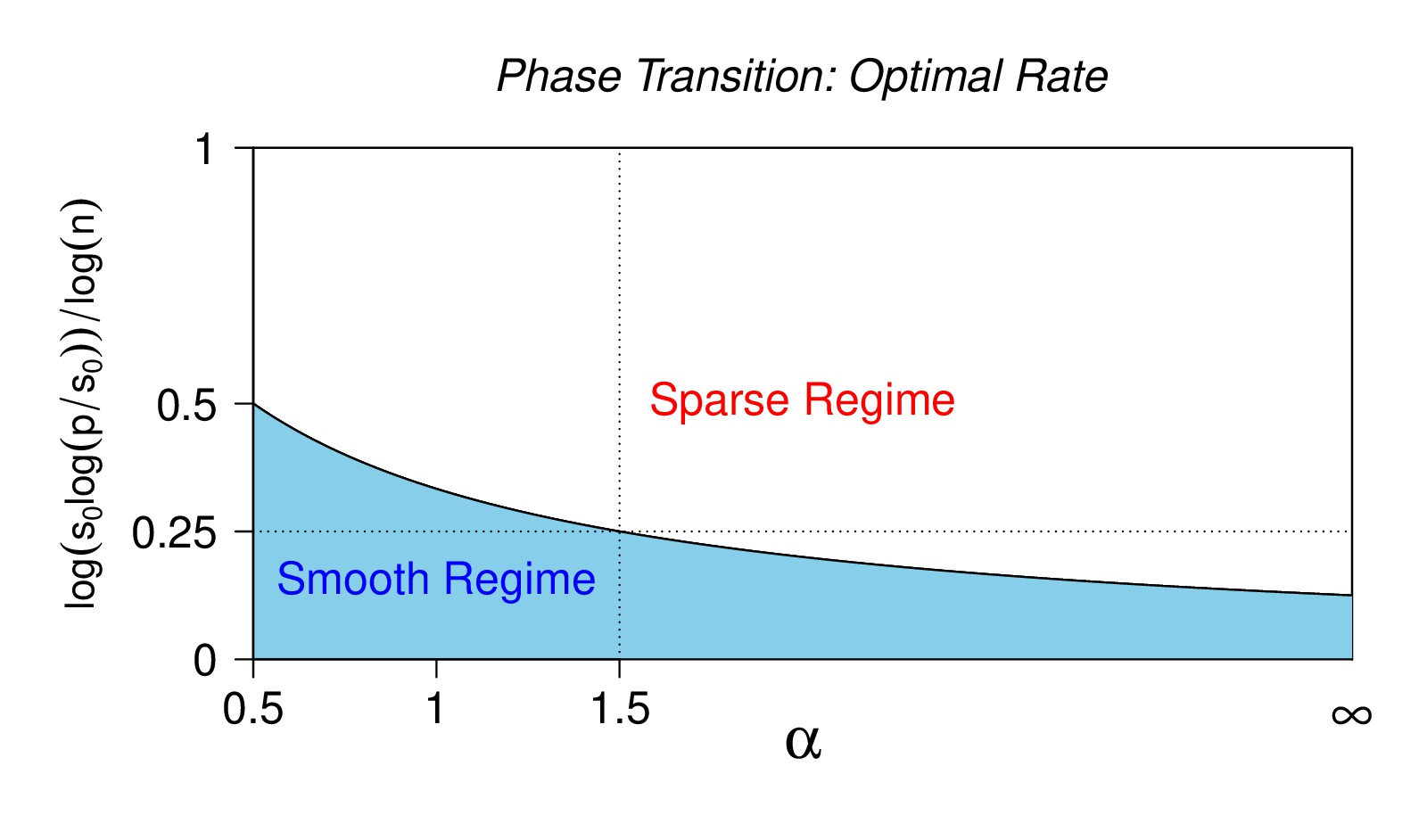# Michael Levine

## Mathematical Statistics and Data Science

Home » ProjectsNonparametric latent variable models

The nonparametric latent variable models are used in many practically important areas of science, such as climate modeling, speech recognition, establishing a differential diagnosis for complex medical conditions, and many others. These models include, as special cases, the well-known density mixtures, HMMs (Hidden Markov Models), mixtures of experts (also called mixtures of regressions), and a number of other useful and practically important models. From the methodological viewpoint, in many cases, the parametric form of components of such a model selected by practicing statisticians is rather arbitrary. Moreover, if one wants to test such a parametric assumption, a nonparametric model has to be considered. It is an interesting fact that estimation for some of these models can be thought of Tikhonov type regularization problems in Banach spaces. This concept is somewhat unusual from the mathematical statistics viewpoint; however, it leads to a number of interesting algorithms that can be used to estimate components of these models. Moreover, the algorithms proposed always have an explicit objective function and are, therefore, relatively easy to analyze. It turns out that most of these algorithms are EM (Expectation-Maximization) type algorithms and their empirical performance is usually quite good. One interesting application area of these algorithms is the economic game theory. There, they have been used to estimate components of the so-called beauty contest auctions. Beauty contest auctions are auctions where the buyer of goods/services does not have to disclose rules he/she follows in selecting auction winners. Such auctions are often held online when looking for freelancers. One of the main problems in this area is the issue of large sample behavior for this class of algorithms. As is common in statistics, it is desirable to find out how close the solution of the problem to the true one when the number of observations is sufficiently large. This is rather different from investigating how the algorithmic solution behaves when the number of iterations increases. Currently, Professor Levine's Ph.D. student, Zhou Shen, is working on a specific problem of estimating a two-component semiparametric density mixture with one known component. Such a model has wide applicability in controlling the rate of false positives in multiple testing; it is also useful when contamination problems, such as commonly encountered in astronomy, have to be studied.Functional estimation in semiparametric models

The function estimation is of profound importance in many applications. Originally, it developed from interest in signal estimation and transmission; today, other areas of application, such as image analysis, are of at least equal interest. Many semiparametric models containing a functional component are very important in econometrics. In a typical econometrics application, estimation of a conditional mean or a conditional quantile is needed where the dependence on covariates can be parameterized for some, but not all, predictors. Those predictors whose influence on the response cannot be parameterized become an argument of the functional component; its estimation has commonly been considered less important than the estimation of parametric part, but now it has become an important issue in its own right. Our current research interest lies mostly in rigorous approach to estimation of functional components in semiparametric models. Viewed from the decision-theoretic viewpoint, such an approach requires establishing minimax convergence rates for estimators of a given functional component as well as constructing estimators adaptive over a wide range of suitable functional classes and distributions of the parametric design part. Compared to functional estimation in nonparametric regression, this area has not been explored as deeply. An additional question of interest rises when a researcher has some control over the choice of covariates to be included in the argument in the function. The optimal choice of such components is a problem from a semiparametric experimental design that has been scarcely explored before.Density mixtures

TBDTime Series

TBD